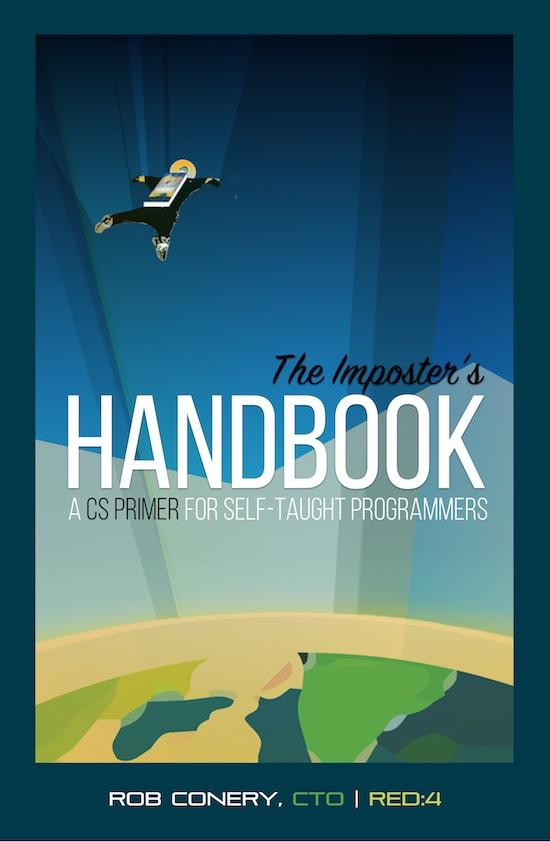# aliquot

## < a quantity that can be divided into another a whole number of time />

Here is a little gem that I came across a few weeks ago, thanks to Twitter:
Conery, R., The Imposter's Handbook (2016), 111 Jackson, Seattle WA 96714: Big Machine, Inc.The associated website on Github contains code in various languages (mainly JS).

Out of curiosity I bought the electronic version, and the PDF is nicely typesetted by the way (except for mathematical equations which are rendered using an ugly and largish font). This was a great read, and I noticed that the author has other books on his shop.1

I'm a self-taught developer and for most of my career I've learned what was required to get the job done. When conversations veered toward core concepts, I disengaged.

I decided to change all of this in 2014. I sat down and looked up all of the topics that a typical CS degree covers and then I dove in. Half way through, I decided to write a book about what I was learning. — Rob Conery

“What does it mean to compute something? How do you tell that to a machine?” (p.7) This reminds me of Donald Knuth about literate programming, but this has nothing to do with the book itself, nor this particular quote:

Let us change our traditional attitude to the construction of programs. Instead of imagining that our main task is to instruct a computer what to do, let us concentrate rather on explaining to human beings what we want a computer to do. — Donald E. Knuth

I learned about Charles Babbage who designed the “difference engine”, which was the first mechanical computer, and then the “analytical engine”, featuring a Turing-complete programming language actionable by punch cards. This was before Ada Lovelace entered the scene and got credited for the very first algorithm on Bernoulli numbers, but see this Wolfram interactive notebook: Ada Lovelace, Bernoulli and the Gauss Schoolboy Problem.

This book is definitively written with elegance. Each chapter begins with a little anecdote. For the first chapter it was an application of prime number theory to a prey and predator model. In the next chapter on complexity, the author discusses how to decide in a rationale and collegial manner on where to go to take a drink altogether (say, $n$ friends), and how naive or more elaborated voting schemes behave as $n$ goes larger and larger. The chapter on complexity also discusses NP-complete problems, like the knapsack and bin packing, finding clique in a graph, or the traveling salesman.

What is particular to this book is that everything is exposed in layman terms, without sacrificing important aspects or more formal details of computer science. For example, consider the chapter on complexity or lambda calculus.2 Except for three or four simple equations, there is no math at all, nor any formal proof. However, one get the sense of how important P and NP problems are in designing algorithms or computer applications. Likewise, the author makes extensive use of Javascript to illustrate various concepts such as lambda expression or Big O notation, e.g., using the Y combinator to compute Fibonacci numbers that you can try right into an online REPL:

let Y = f => (x => x(x))(x => f(y => x(x)(y)));
let fib = f => n => n <= 1 ? n : f(n-1) + f(n-2);
let yFib = Y(fib);
yFib(10);


Another interesting aspect of the book is the fact that the author deliberately chose to focus on concepts rather than tools or algorithmic details. This means that he will present the main data structures (array, hash, linked list, etc.) or the complexity of some common operations (e.g., iterations that use divide and conquer are always $\mathcal{O}(n\log{}n)$), but he will not delve into the details of a particular implementation (although they are all sound and nicely illustrated) or programming language, as in Kumar, S. Clojure High Performance Programming (Packt Publishing, 2013), for instance. Each time, he find a way to link to historical details, like the Königsberg bridges in the case of graph structure. Finally, some of the illustrations are gorgeous. The one on quick sort deserves some mention.

I still have the last chapters to read, but surely this brief sketch will let you consider to read the book or not.

♪ Young Marble Giants • Colossal Youth

1. Note that the author's brother, John S. Conery, also wrote a book: Explorations in Computing. ↩︎

2. A very nice introduction to lambda calculus is also available in Allen, C., & Moronuki, J. (2016). Haskell programming from first principles. ↩︎